#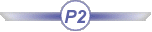This scenario shows how to create a Knowledge Pattern. It is based on ARM and on the new Knowledge directories structure. For more information, see CATIA Infrastructure User's Guide: Advanced Tasks: Using Knowledgeware Capabilities: Managing Knowledge Applications Resources.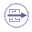To create a Knowledge Pattern, you have to: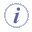To perform this scenario, you will need the following files:
 PktknowledgepatternDatum.CATPart This document will contain the created Knowledge Pattern and the instantiated User Features. PktTemplate.CATPart This document contains the 3 User Features that will be instantiated from the Knowledge Patterns. UserFeature2 is instantiated from the first Knowledge Pattern. Its inputs are 2 points. UserFeature3 is instantiated from the first Knowledge Pattern. Its inputs are 2 points. UserFeature4 which is instantiated from the second Knowledge Pattern. Its inputs are 3 lines. Udf2.CATGScript This file contains the information concerning the User Feature2. Udf3.CATGScript This file contains the information concerning the User Feature3. Udf4.CATGScript This file contains the information concerning the User Feature4. PktARMcatalog3.catalog This document is the ARM catalog referencing the physical resources used in this scenario.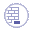Before performing this scenario, make sure that you have:
• Selected the directory that contains the Udf2.CATGScript, Udf3.CATGScript and the Udf4.CATGScript in the Reference Directory For Types field in the Tools>Options>General>Parameter and Measures>Knowledge Environment tab.
• Selected the Architect Resources Creation Path in the Tools>Options>General>Parameter and Measures>Knowledge Environment tab. Remember that the directory selected here can only be the ``` knowledge``` directory of the Knowledge directories structure. For more information about this structure, see CATIA Infrastructure User's Guide: Advanced Tasks: Using Knowledgeware Capabilities: Managing Knowledge Applications Resources.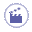## Save the Required Data into the New Directories Structure

1. Save the provided data in the appropriate directories: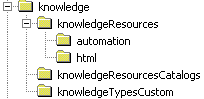Save PktknowledgepatternDatum.CATPart and PktTemplate.CATPart in the `CATKnowledgePath\knowledge\knowledgeResources` directory. Save Udf2.CATGScript, Udf3.CATGScript, Udf4.CATGScript in the `knowledgeTypesCustom` directory referenced in the Reference Directory For Types. Save PktARMcatalog3.catalog in the ``` CATKnowledgePath\knowledge\knowledgeResourcesCatalogs``` directory.

## Create the Parameters Driving the Patterns

1. Open the PktknowledgepatternDatum.CATPart file.

2. Create the parameter indicating if odd index instances are typed udf1 or udf2:

 Click the Formula icon (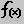). The Formula editor opens. In the New parameter of type combo box, select Integer and Multiple Values, and In the Edit name or value of the current parameter field, select Integer.1 and enter Config. Click New parameter of type. A dialog box displays. Enter 0 and 1 and click OK to validate.
3. Create the parameter indicating if datum features based on the User Features are to be generated. If this parameter is set to True, datum features are generated and User Features removed.

 In the New parameter of type combo box, select Boolean, and In the Edit name or value of the current parameter field, select Boolean.1 and enter GenerateDatum. Click New parameter of type.

## Create the List Grouping the Points

2. Click the List icon (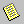). The List Edition dialog box opens. Click OK. Rename the created list List.1 into Points.

3. Click the Formula icon (). The Formula editor opens.

4. Click the Points list in the specification tree and click Add Formula.

5. Enter the following formula and click OK twice to validate: `PointsBody .Query("Point", "")`.

## Create the Knowledge Pattern Generating the Lines

1. From the Start>Knowledgeware menu, select the Product Knowledge Template workbench.

2. Click the Create a Knowledge Pattern icon (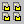). The Knowledge Pattern Editor opens.

3. Enter the following code in the editor or copy/paste the code contained in this file:

 `let obj1 (Udf2) ` (1)``` let obj2 (Udf3) let p (Point) let p2 (Point) let list (List) let i (Integer) let j (Integer) let k (Integer) let m (Integer) let line (Line) let line2 (Line) set list = Points i = 1 k = 1 m = Config For i while i <= list.Size()              {                          j = 1                          For j while j <= list.Size()                          {                                       if i < j                                       {                                                 set p = list.GetItem(i)                                                 set p2 = list.GetItem(j)                                                 if m == 0                                                 {                                                 set obj1 = CreateOrModifyTemplate("PktARMcatalog3|UserFeature2", PartBody , Lines, k)                                                  obj1.Point.1 = p                                                  obj1.Point.2 = p2                                                  obj1.Name = "UdfType1_" + ToString(k)                                                  EndModifyTemplate(obj1)                                       }                                       else                                                 {                                                 set obj2 = CreateOrModifyTemplate("PktARMcatalog3|UserFeature3", PartBody ,Lines, k)                                                 obj2.Point.1 = p                                                 obj2.Point.2 = p2                                                 obj2.Name = "UdfType2_" + ToString(k)                                                 EndModifyTemplate(obj2)                                                 }                                                 k = k + 1                                                 m = 1 - m                                        }                                        j = j +1                           }                           i = i+ 1                  }                   i = 1                   if GenerateDatum == True (```2)```                   {                                 for i while i <= Lines.Size()                                 {                                             line = CreateOrModifyDatum("Line", PointsBody, Lines2, i) ```(3)```                                             set line2 = Lines.GetItem(i)                                             line = line2                                             line.Color = line2.Color                                             i = i + 1                                   }                                    for i while Lines.Size() > 0                                             {                                                  Lines.RemoveItem(1)```(4)```                                              }                  }```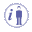In the script above, you first declare the local variables (1). The list points the Points list. The list is parsed, a couple of points is retrieved and the User Feature (line) is instantiated on these 2 points. This operation is performed for all points. If `GenerateDatum == True `(2)```, ```datum lines based on the lists created previously are generated using the `CreateOrModifyDatum` method (3) and the the lines previously created are removed (4).
1. Click Add. List.1 = 0 elements is displayed in the Knowledge Pattern Lists field.

2. Click List.1 and replace it with Lines in the Name field.

3. Click Add. List.2 = 0 elements is displayed in the Knowledge Pattern Lists field.

4. Click it and replace it with Lines2 in the Name field.

5. In the Mode combo box, select Manual execution. Click OK when done.

## Create the Knowledge Pattern Generating the Surfaces

1. Click the Create a Knowledge Pattern icon (). The Knowledge Pattern Editor opens.

2. Enter the following code in the editor or copy/paste the code contained in this file:

 ```let obj (Udf4) let line1 (Udf3) let line2 (Udf3) let line3 (Udf3) let p1 (Point) let p2 (Point) let p3 (Point) let p4 (Point) let p5 (Point) let p6 (Point) let p7 (Point) let i (Integer) let j (Integer) let k (Integer) let m (Integer) i = 1 j=1 k = 1 m=1 For i while i <= Lines.Size() {              j = 1              For j while j <= Lines.Size()              {                           k = 1                           if i < j                           {                                     set line1 = Lines.GetItem(i)                                     set line2 = Lines.GetItem(j)                                     set p1 =  line1.GetAttributeObject("Point.1")                                     set p2 =  line1.GetAttributeObject("Point.2")                                     set p3 =  line2.GetAttributeObject("Point.1")                                     set p4 =  line2.GetAttributeObject("Point.2")                                     if p1 == p3 or p1 == p4 or p2 == p3 or p2 == p4                                     {                                                 if p1 == p3 or p1 == p4                                                             p7 = p1                                                 else                                                             p7 = p2                                                  For k while k <= Lines.Size()                                                  {                                                               if j < k                                                               {                                                                   set line3 = Lines.GetItem(k)                                                                   set p5 = line3.GetAttributeObject("Point.1")                                                                   set p6 = line3.GetAttributeObject("Point.2")                                                                   if (p5 == p1 or p5 == p2 or p5 == p3 or p5 == p4)and (p6 == p1 or p6 == p2                                                                   or p6 == p3 or p6 == p4)                                                                   {                                                                   set obj =                                                                   CreateOrModifyTemplate("PktARMcatalog3|UserFeature4", PartBody,                                                                   Surfaces, m)                                                                   obj.Line.1 = line1                                                                   obj.Line.2 = line2                                                                   obj.Line.3 = line3                                                                   EndModifyTemplate(obj)                                                                   m = m +1                                                                   }                                                                 }                                                                 k = k + 1                                                   }                                  }                         }                          j = j +1 }```In the script above, you first declare the local variables. you retrieve triangles made of 3 points and 3 lines and you instantiate UserFeature4 which is a surface using the `CreateOrModifyTemplate `method.
1. Click Add. List.1 = 0 elements is displayed in the Knowledge Pattern Lists field.

2. Click List.1 and replace it with Surfaces in the Name field. Click OK when done.

3. Right-click Knowledge Pattern.1 and select Knowledge Pattern.1 object>Execute. The lines are created.

4. Right-click Knowledge Pattern.2 and select Knowledge Pattern.2 object>Execute. The surfaces are created. Click here to open the Part containing the Knowledge Patterns.